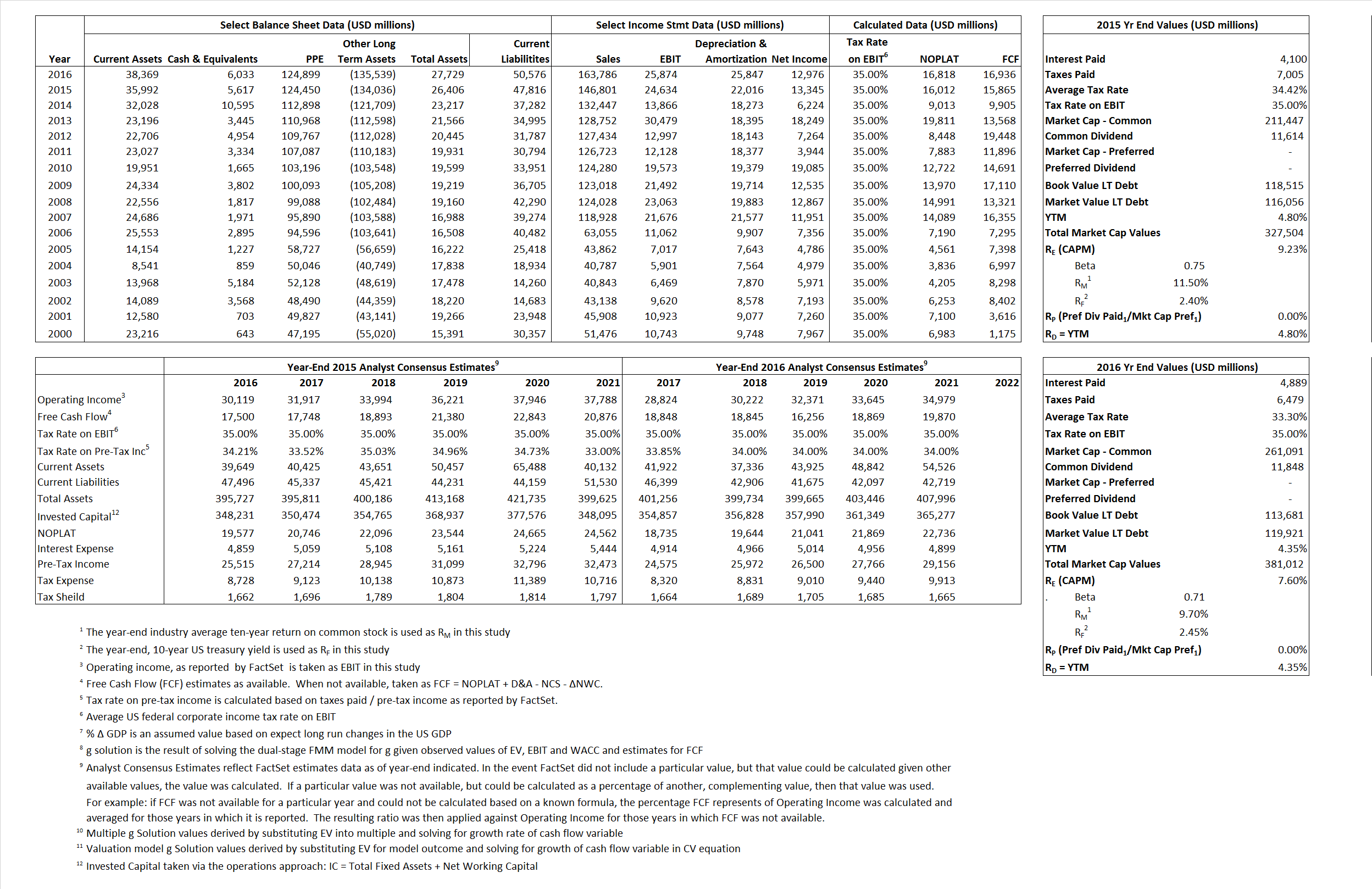# AT&T

## Analyst Listing

The following analysts provide coverage for the subject firm as of May 2016:

 Broker Analyst Analyst Email Drexel Hamilton Barry M. Sine bsine@drexelhamilton.com Cowen & Company Colby Synesael colby.synesael@cowen.com FBR Capital Markets & Co David Dixon ddixon@fbr.com Raymond James Frank G. Louthan IV frank.louthan@raymondjames.com William Blair James D. Breen jbreen@williamblair.com DA Davidson James Moorman jmoorman@dadco.com Buckingham Research James Ratcliffe jratcliffe@buckresearch.com Scotiabank GBM Jeffrey Fan jeff.fan@scotiabank.com Nomura Research Jeffrey Kvaal jeffrey.kvaal@nomura.com Wells Fargo Securities Jennifer M. Fritzsche jennifer.fritzsche@wellsfargo.com RBC Capital Markets Jonathan Atkin jonathan.atkin@rbccm.com Evercore ISI Jonathan Schildkraut schildkraut@evercoreisi.com Credit Suisse Joseph Mastrogiovanni joseph.mastrogiovanni@credit-suisse.com Deutsche Bank Research Matthew Niknam matthew.niknam@db.com Pacific Crest Securities-KBCM Michael G. Bowen mbowen@pacific-crest.com Jefferies Mike McCormack mmccormack@jefferies.com Bernstein Research Paul de Sa paul.desa@bernstein.com Oppenheimer Timothy Horan tim.horan@opco.com BTIG Walter Piecyk wpiecyk@btig.com

## Primary Input Data## Derived Input Data

### Equational Form

Net Operating Profit Less Adjusted Taxes NOPLAT  16,012 16,818$NOPLAT\, =\, EBIT\, x\, (1 \,-\, Avg \,\,Tax\,\, Rate\,\, on\,\, EBIT)$
Free Cash Flow FCF 15,865 16,936$FCF\,=NOPLAT\,+\,Non-Cash\,Expenses-\Delta NWC\,-\,NCS$
Tax Shield TS 1,411 1,628$TS\,=\,Interest\,\,Paid\,\,x\,\, Avg \,\,Tax\,\,Rate\,\, on\,\, Pre-Tax\,\, Income$
Invested Capital IC (21,410) (22,847)$IC\,=\,Fixed\,\,Operating\,\,Assets\,\,+\,\,Net\,\, Working\,\, Capital$
Return on Invested Capital ROIC -74.79% -73.61%$ROIC\,=\,\frac { NOPLAT }{ IC }$
Net Investment NetInv 14,671 24,410$NetInv\,=\,{ {IC}_{1}}-{{IC}_{0}}+Depreciation$
Investment Rate IR 91.62%  145.14%$IR\,=\,\frac {NetInv}{NOPLAT}$
Weighted Average Cost of Capital
WACCMarket 7.07% 6.12%$WACC\,=\,\frac { E }{ V } { R }_{ E }\,+\,\frac { P }{ V } { R }_{ P }\,+\,\frac { D }{ V } { R }_{ D }\left( 1- Avg\,\, Tax\,\,Rate\,\,on\,\,Pre-Tax\,\,Income \right)$
WACCBook  5.53%    5.52%
Enterprise value
EVMarket 321,887 374,979$EV\,=\,Market\,\,Cap\,\,Equity\,+\,\,Long\,\,Term\,\,Debt\,-\,Cash$
EVBook  323,929  368,739
Long-Run Growth
g = IR x ROIC
-68.52%  -106.84% Long-run growth rates of the income variable are used in the Continuing Value portion of the valuation models.
g = %$\Delta$ GDP  2.50%     2.50%
Margin from Operations M 16.78%    15.80%$M\,\,=\,\,\frac{EBIT}{SALES}$
Depreciation/Amortization Rate D 47.19%    49.97%$D\,\,=\,\,\frac{D+A}{EBITDA}$

## Valuation Multiple Outcomes

The outcomes presented in this study are the result of original input data, derived data, and synthesized inputs.

### model g solution

12/31/2015 12/31/2016 12/31/2015 12/31/2016 12/31/2015 12/31/2016

EV/SALES$\frac {EV}{Sales} \,= \,\frac{ROIC\, -\, g}{ROIC\,(WACC\,-\,g)}\,(1\,-\,T)\,(M)$

2.19  2.29  1.97%  1.54%  2.23%  2.67%

EV/EBITDA$\frac {EV}{EBITDA} \,= \,\frac{ROIC\, -\, g}{ROIC\,(WACC\,-\,g)}\,(1\,-\,T)\,(1\,-\,D)$

6.90 7.25 1.97% 1.54% 2.23% 2.67%

EV/NOPLAT$\frac {EV}{NOPLAT} \,= \,\frac{ROIC\, -\, g}{ROIC\,(WACC\,-\,g)}$

20.10 22.30 1.97% 1.54% 2.23% 2.67%

EV/FCFOPS$\frac {EV}{FCF_{OPS}} \,= \,\frac{ROIC\, -\, g}{ROIC\,(WACC\,-\,g)}\,(1\,-\,T)$

20.29 22.14 1.97% 1.54% 2.23% 2.67%

EV/EBIT$\frac {EV}{EBIT} \,= \,\frac{ROIC\, -\, g}{ROIC\,(WACC\,-\,g)}\,(1\,-\,T)$

13.07 14.49 1.97% 1.54% 2.23% 2.67%

EV/IC$\frac {EV}{IC} \,= \,\frac{ROIC\, -\, g}{WACC\,-\,g}$

-15.03 -16.41 1.97% 1.54% 2.23% 2.67%